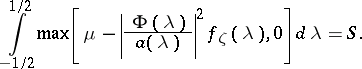# Gaussian channel

(diff) ← Older revision | Latest revision (diff) | Newer revision → (diff)

A communication channel whose transition function determines a conditional Gaussian distribution. More precisely, a communication channel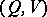is called a Gaussian channel on the finite interval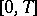if the following conditions hold: 1) the spaces of values of the input and output signals,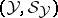and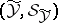, are spaces of real-valued functions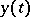and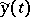,, with the usual-algebras of measurable sets (that is, the input and output signals of a Gaussian channel are given by stochastic processes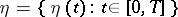and, respectively); 2) for any fixedthe transition function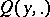of the channel determines a conditional Gaussian distribution (one says that a collection of random variables has a conditional Gaussian distribution if every finite subfamily has a conditional finite-dimensional normal distribution with second moments that are independent of the conditioning); and 3) the restrictionis imposed only on the second moment of the random variable.

An example of a Gaussian channel onis a channel whose input signal is given by a stationary random sequence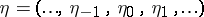and whose output signal is the stationary random sequence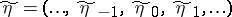, obtained according to the formulaswhereis a stationary Gaussian random sequence independent ofwith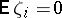,and with spectral density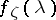,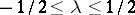. The restriction on the input signal has the formwhere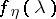is the spectral density of,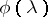is some function andis a constant. The capacity of such a channel is given by the formula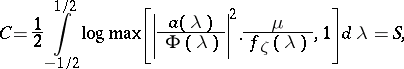where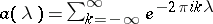andis determined by the equation